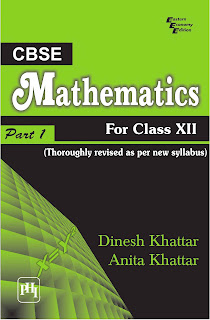ANALYSICS MATHEMATICS

# CBSE Mathematics The matics for Class XII Part I Thoroughly Revised as Per New CBSE Syllabus PDF Free DownloadStrictly as per the new CBSE course structure and NCERT guidelines, this thoroughly revised and updated textbook is meant for class XII of senior secondary schools (under the 10 + 2 pattern of education). The subject matter of this book is presented in a very systematic and logical manner. Every effort has been made to make the contents as lucid as possible so that the beginners will grasp the fundamental concepts in an unambiguous manner. KEY FEATURES Large number of solved examples to understand the subject. Categorization of problems under: Level of Difficulty A (Cover the needs of the students preparing for CBSE exams) Level of Difficulty B (Guide the students for engineering entrance examinations). A Smart Table at the beginning of each chapter to decide the relative importance of topics in the CBSE exam. Problem Solving Trick(s) to enhance the problem solving skills. A list of Important Formulae at the beginning of the book. Besides this, each chapter is followed by a Chapter Test and an exercise in which the questions from the CBSE papers of previous years are provided. Working hints to a large number of problems are given at the end of each and every exercise. In a nut shell, this book will help the students score high marks in CBSE, and at the same time build a strong foundation for success in any competitive examination.